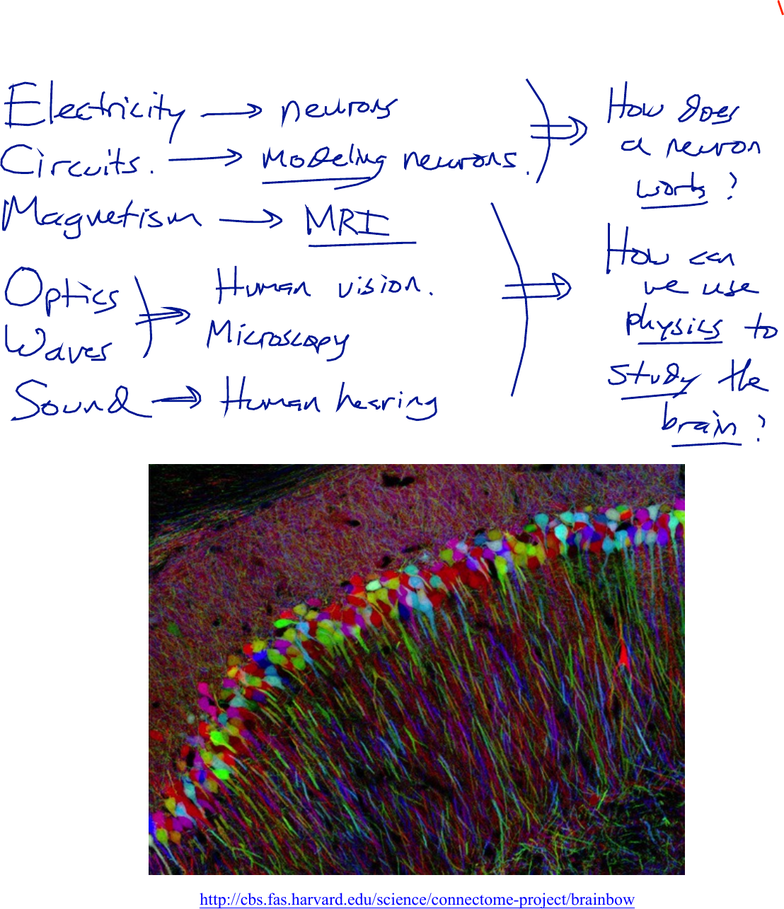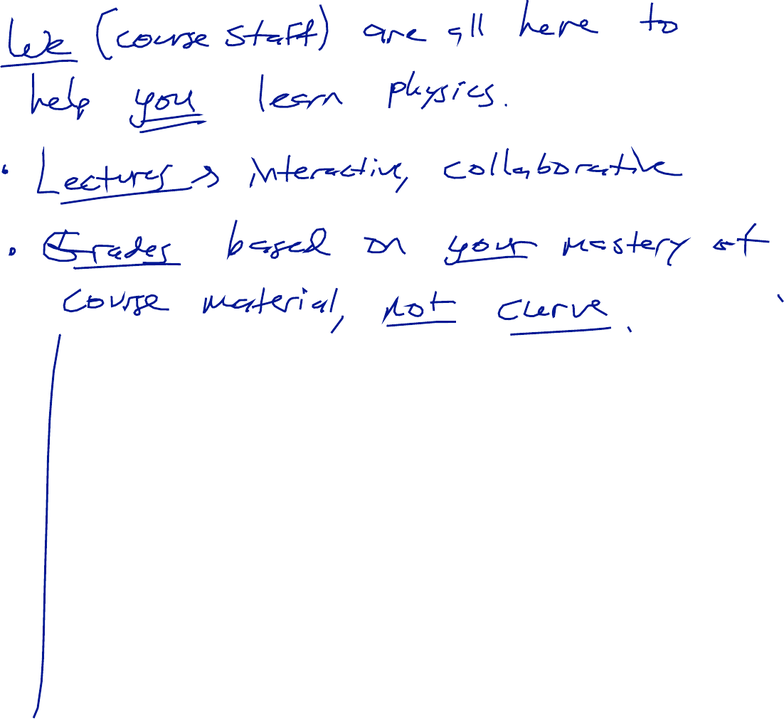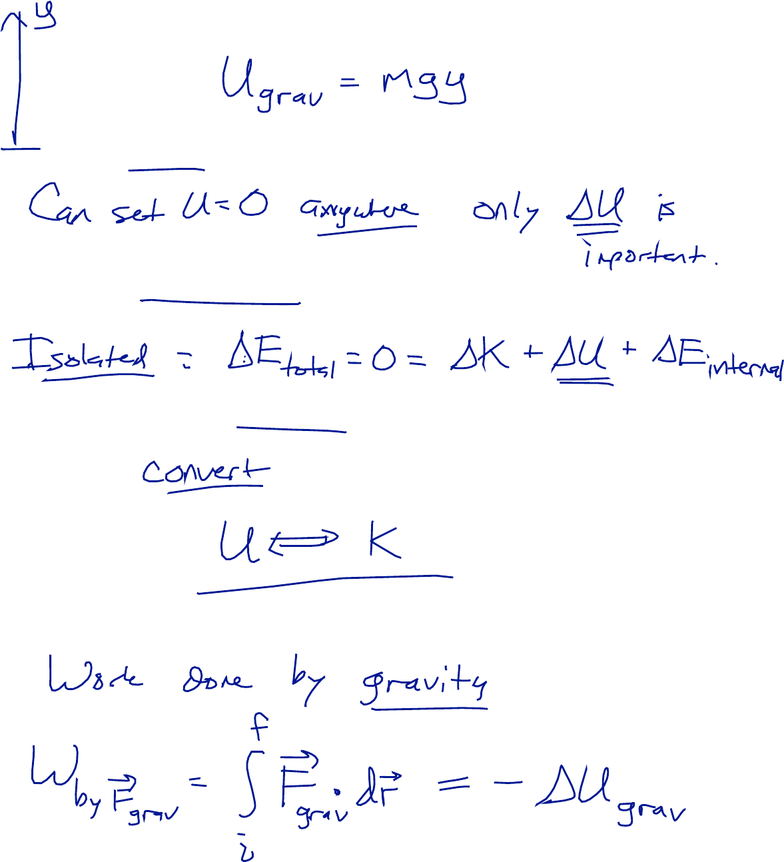Study Guides (400,000)
US (230,000)
Harvard (700)
(20)
Final

# Physical Sciences 3 Lecture 1: Lecture 1aExam

Department
Physical Sciences
Course Code
Physical Sciences 3
Professor
Roxanne Guenette
Study Guide
Final

This preview shows pages 1-3. to view the full 11 pages of the document.Physical Sciences 3: Lecture 1a
January 29, 2019
1
Welcome to Physical Sciences 3
What will we be learning this semester?
Why are we learning all of this stuff?
http://cbs.fas.harvard.edu/science/connectome-project/brainbow

Only pages 1-3 are available for preview. Some parts have been intentionally blurred.Physical Sciences 3: Lecture 1a
January 29, 2019
2
Introduction
Our course philosophy and approach to teaching:
Learning objectives: After this lecture, you will be able to…
1. Define the gravitational potential at any point in space, and determine the gravitational
potential energy of a mass placed at that point.
2. Describe the concept of a field and give some examples of scalar fields.
3. Define the gradient of a field and give some examples of how to find the gradient of a
field.
4. Calculate the gravitational force exerted on a mass, given the gravitational potential.
5. Define the electric potential at any point in space, and calculate the electric potential
energy of a charge placed at that point.
6. Calculate the electrostatic force exerted on a charge, given the electric potential.
7. Describe how you could create a linear potential gradient inside a wire.
8. Calculate the electrostatic force on a charge in a linear potential gradient.

Only pages 1-3 are available for preview. Some parts have been intentionally blurred.Physical Sciences 3: Lecture 1a
January 29, 2019
3
Review: Gravitational Potential Energy
Recall from last semester: a mass at a point y above the earth’s surface has gravitational
potential energy:
Note that the value of the potential energy itself is not physically meaningful; only
differences in potential energy can be measured:
Why is the concept of gravitational potential energy useful?
- Conservation of energy
- Relationship with kinetic energy
- Relationship with work